Home > AC > Chapter 11 > Lesson 11.1.1 > Problem11-13

11-13.
1. Add, subtract, multiply, or divide the following rational expressions. Simplify your answers if possible. Homework Help ✎

1.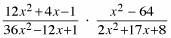2.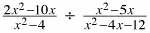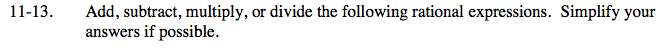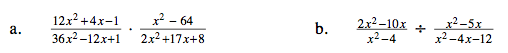Factor the expressions, then multiply them.

$\frac{\left (2x+1 \right )\left ( 6x+1 \right )}{(6x-1)(6x-1)}\cdot \frac{\left (x-8 \right )\left ( x+8 \right )}{(2x+1)(x+8)}$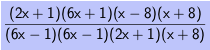Look for factors that make 1.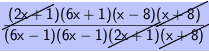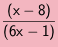Change division to multiplication, then see part (a).